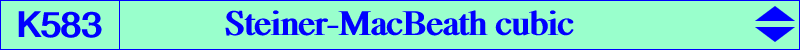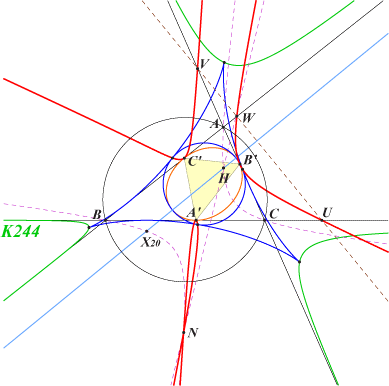too complicated to be written here. Click on the link to download a text file.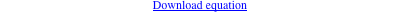X(14249), X(42753), X(42757) X(58258) → X(58264), X(58280) → X(58284) imaginary foci of the MacBeath inconic A', B', C' : cevian points of X(264), the contacts of the MacBeath conic with the sidelines of ABC U, V, W : traces of the perpendicular at X(403) to the Euler line N = X(14249) = X(3)X(107) /\ X(4)X(51), a node on the cubic contacts of the nine point circle with the Steiner deltoid H3 and, more generally, of the incircle of any deltoid inscribed in ABC with the deltoidThe locus of the contacts of the deltoids inscribed in ABC with their incircles is the Steiner-MacBeath cubic K583. The locus of their cusps is the Erhmann-MacBeath cubic K244. K583 is a nodal cubic inscribed in ABC with contacts those of the MacBeath conic. Recall that this latter conic is inscribed in ABC, with center X(5), perspector X(264), real foci O and H. Its imaginary foci lie on K583 and on the perpendicular bisector of OH. The third common point is P3, barycentric product of X(850) and X(3163), on the lines {X4,X520}, {X5,X523}, {X113,X133}. The node N is the barycentric product of X(2052) and X(20). The trilinear polar of X(2052) is the perpendicular at X(403) to the Euler line and meets the cubic at U, V, W on the sidelines of ABC. N lies on several lines such as X(3)X(107), X(4)X(51), X(253)X(264), etc, and also on the rectangular circum-hyperbola that contains X(20). Since all the incircles of the deltoids have their center on the perpendicular bisector of OH, there are infinitely many equilateral triangles inscribed in K583 and obviously their centers lie on this same line. The figure below shows three of these triangles, namely those having a vertex at U, V, W.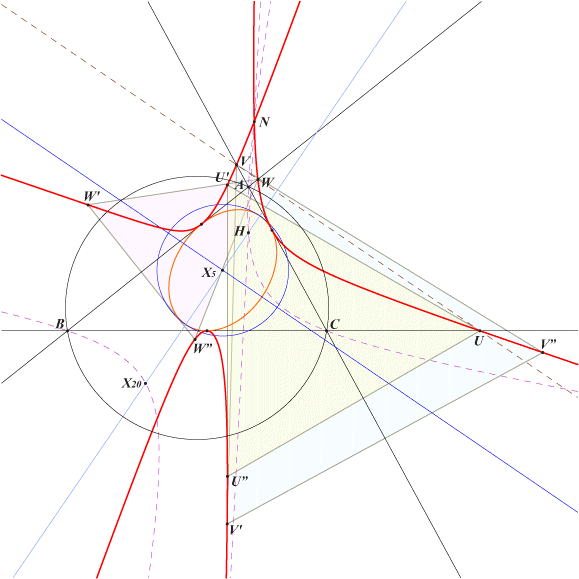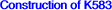Let M be a variable point on (O) and M' its isotomic conjugate (on the de Longchamps axis). The infinite point of the Simson line of M has its barycentic square S on the Steiner inellipse. The barycentric product P of M' and S is a point on K583. *** A few other centers on K583 b^2 (b-c)^2 c^2 (b+c)^2 (-2 a^4+a^2 b^2+b^4+a^2 c^2-2 b^2 c^2+c^4) : : , SEARCH = 3.314664587240124 a^4 (b-c)^2 (b+c)^2 (a^2 b^2-b^4+a^2 c^2-c^4) : : , SEARCH = 1.861404377588349 a^4 (b-c) (b+c) (a^2 b^2-b^4+a^2 c^2-c^4)^2 : : , SEARCH = 5.733279192272652 b^2 (b-c) c^2 (-2 a^3+a^2 b+b^3+a^2 c-b^2 c-b c^2+c^3)^2 : : , SEARCH = 21.58818657813396 b^2 (b-c)^2 c^2 (-2 a^3+a^2 b+b^3+a^2 c-b^2 c-b c^2+c^3) : : , SEARCH = 2.982856123440201 a (b-c)^2 (a b-b^2+a c-c^2) (a^2-2 a b+b^2-2 a c+c^2)^2 : : , SEARCH = -0.9360570383765106 b^2 (b-c)^2 c^2 (-3 a+b+c)^2 (-2 a+b+c) : : , SEARCH = -2.464628143000415 b^2 (b-c) c^2 (b+c) (-2 a^4+a^2 b^2+b^4+a^2 c^2-2 b^2 c^2+c^4)^2 : : , SEARCH = 45.00906052671399 b^2 (b-c)^2 c^2 (b+c)^2 (-5 a^2+b^2+c^2)^2 (-2 a^2+b^2+c^2) : : , SEARCH = -1.761444221187371 b^2 (b-c)^2 c^2 (-a b-a c+2 b c) (-3 a^3-a b^2-2 a b c+2 b^2 c-a c^2+2 b c^2)^2 : : , SEARCH = -3.980426093372173 b^2 (b-c)^2 c^2 (b+c) (-a^2+b c) (-a^3-a^2 b-a^2 c-a b c+b^2 c+b c^2)^2 : : , SEARCH = -3.217862499195757 b^2 (b-c)^2 c^2 (-2 a^3+b^3+c^3) (-2 a^2 b-a b^2+b^3-2 a^2 c+a b c-a c^2+c^3)^2 : : , SEARCH = -1.138334987571258 b^2 (b-c)^2 c^2 (b+c)^2 (-2 a^4+b^4+c^4) (-2 a^4-3 a^2 b^2+b^4-3 a^2 c^2+c^4)^2 : : , SEARCH = -0.6145344301149047 b^2 (b-c) c^2 (b^2+b c+c^2) (-2 a^5+a^2 b^3+b^5-b^3 c^2+a^2 c^3-b^2 c^3+c^5)^2 : : , SEARCH = 235.747683379761 b^2 (b-c) c^2 (b+c) (b^2+c^2) (-2 a^6+a^2 b^4+b^6-b^4 c^2+a^2 c^4-b^2 c^4+c^6)^2 : : , SEARCH = -111.8981450368373 b^2 (b-c) c^2 (b+c) (-5 a^2+b^2+c^2) (-2 a^2+b^2+c^2)^2 : : , SEARCH = -12.43554213938931